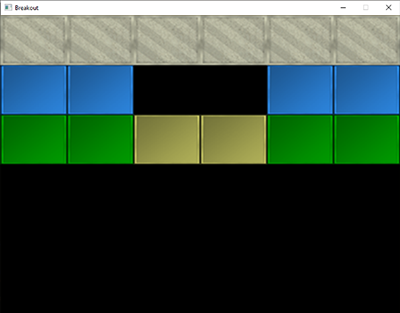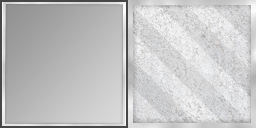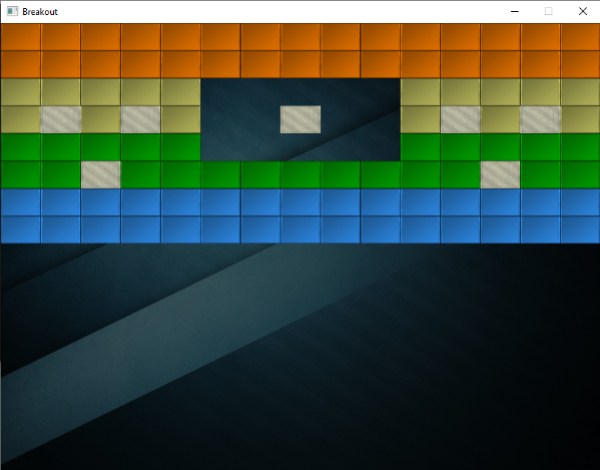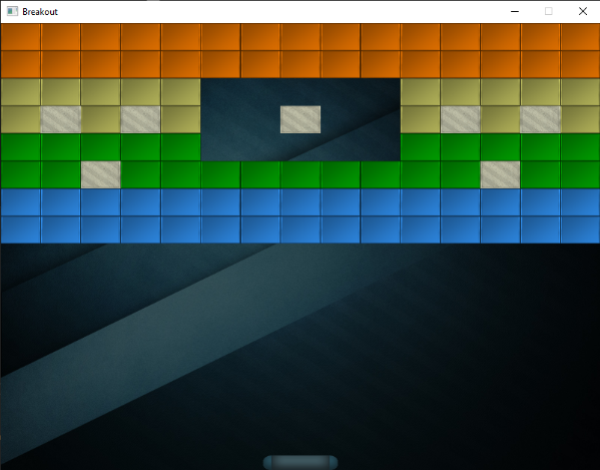If you're running AdBlock, please consider whitelisting this site if you'd like to support LearnOpenGL; and no worries, I won't be mad if you don't :)

# Levels

Breakout is unfortunately not just about a single happy green face, but contains complete levels with a lot of playfully colored bricks. We want these levels to be configurable such that they can support any number of rows and/or columns, we want the levels to have solid bricks (that cannot be destroyed), we want the levels to support multiple brick colors, and we want them to be stored externally in (text) files.

In this chapter we'll briefly walk through the code of a game level object that is used to manage a large amount of bricks. We first have to define what an actual brick is though.

We create a component called a game object that acts as the base representation of an object inside the game. Such a game object holds state data like its position, size, and velocity. It holds a color, a rotation component, whether it is solid and/or destroyed, and it also stores a Texture2D variable as its sprite.

Each object in the game is represented as a GameObject or a derivative of this class. You can find the code of the GameObject class below:

A level in Breakout consists entirely of bricks so we can represent a level by exactly that: a collection of bricks. Because a brick requires the same state as a game object, we're going to represent each brick of the level as a GameObject. The declaration of the GameLevel class then looks as follows:


class GameLevel
{
public:
// level state
std::vector<GameObject> Bricks;
// constructor
GameLevel() { }
void Load(const char *file, unsigned int levelWidth, unsigned int levelHeight);
// render level
void Draw(SpriteRenderer &renderer);
// check if the level is completed (all non-solid tiles are destroyed)
bool IsCompleted();
private:
// initialize level from tile data
void init(std::vector<std::vector<unsigned int>> tileData,
unsigned int levelWidth, unsigned int levelHeight);
};


Since a level is loaded from an external (text) file, we need to propose some kind of level structure. Here is an example of what a game level may look like in a text file:


1 1 1 1 1 1
2 2 0 0 2 2
3 3 4 4 3 3


A level is stored in a matrix-like structure where each number represents a type of brick, each one separated by a space. Within the level code we can then assign what each number represents. We have chosen the following representation:

• A number of 0: no brick, an empty space within the level.
• A number of 1: a solid brick, a brick that cannot be destroyed.
• A number higher than 1: a destroyable brick; each subsequent number only differs in color.

The example level listed above would, after being processed by GameLevel, look like this:The GameLevel class uses two functions to generate a level from file. It first loads all the numbers in a two-dimensional vector within its Load function that then processes these numbers (to create all game objects) in its init function.


void GameLevel::Load(const char *file, unsigned int levelWidth, unsigned int levelHeight)
{
// clear old data
this->Bricks.clear();
unsigned int tileCode;
GameLevel level;
std::string line;
std::ifstream fstream(file);
std::vector<std::vector<unsigned int>> tileData;
if (fstream)
{
while (std::getline(fstream, line)) // read each line from level file
{
std::istringstream sstream(line);
std::vector<unsigned int> row;
while (sstream >> tileCode) // read each word separated by spaces
row.push_back(tileCode);
tileData.push_back(row);
}
if (tileData.size() > 0)
this->init(tileData, levelWidth, levelHeight);
}
}


The loaded tileData is then passed to the game level's init function:


void GameLevel::init(std::vector<std::vector<unsigned int>> tileData,
unsigned int lvlWidth, unsigned int lvlHeight)
{
// calculate dimensions
unsigned int height = tileData.size();
unsigned int width  = tileData.size();
float unit_width    = lvlWidth / static_cast<float>(width);
float unit_height   = lvlHeight / height;
// initialize level tiles based on tileData
for (unsigned int y = 0; y < height; ++y)
{
for (unsigned int x = 0; x < width; ++x)
{
// check block type from level data (2D level array)
if (tileData[y][x] == 1) // solid
{
glm::vec2 pos(unit_width * x, unit_height * y);
glm::vec2 size(unit_width, unit_height);
GameObject obj(pos, size,
ResourceManager::GetTexture("block_solid"),
glm::vec3(0.8f, 0.8f, 0.7f)
);
obj.IsSolid = true;
this->Bricks.push_back(obj);
}
else if (tileData[y][x] > 1)
{
glm::vec3 color = glm::vec3(1.0f); // original: white
if (tileData[y][x] == 2)
color = glm::vec3(0.2f, 0.6f, 1.0f);
else if (tileData[y][x] == 3)
color = glm::vec3(0.0f, 0.7f, 0.0f);
else if (tileData[y][x] == 4)
color = glm::vec3(0.8f, 0.8f, 0.4f);
else if (tileData[y][x] == 5)
color = glm::vec3(1.0f, 0.5f, 0.0f);

glm::vec2 pos(unit_width * x, unit_height * y);
glm::vec2 size(unit_width, unit_height);
this->Bricks.push_back(
GameObject(pos, size, ResourceManager::GetTexture("block"), color)
);
}
}
}
}


The init function iterates through each of the loaded numbers and adds a GameObject to the level's Bricks vector based on the processed number. The size of each brick is automatically calculated (unit_width and unit_height) based on the total number of bricks so that each brick perfectly fits within the screen bounds.

Here we load the game objects with two new textures, a block texture and a solid block texture.A nice little trick here is that these textures are completely in gray-scale. The effect is that we can neatly manipulate their colors within the game-code by multiplying their grayscale colors with a defined color vector; exactly as we did within the SpriteRenderer. This way, customizing the appearance of their colors doesn't look too weird or unbalanced.

The GameLevel class also houses a few other functions, like rendering all non-destroyed bricks, or validating if all non-solid bricks are destroyed. You can find the source code of the GameLevel class below:

The game level class gives us a lot of flexibility since any amount of rows and columns are supported and a user could easily create his/her own levels by modifying the level files.

## Within the game

We would like to support multiple levels in the Breakout game so we'll have to extend the game class a little by adding a vector that holds variables of type GameLevel. We'll also store the currently active level while we're at it:


class Game
{
[...]
std::vector<GameLevel> Levels;
unsigned int           Level;
[...]
};


This series' version of the Breakout game features a total of 4 levels:

Each of the textures and levels are then initialized within the game class's Init function:


void Game::Init()
{
[...]
GameLevel one; one.Load("levels/one.lvl", this->Width, this->Height / 2);
GameLevel two; two.Load("levels/two.lvl", this->Width, this->Height / 2);
GameLevel three; three.Load("levels/three.lvl", this->Width, this->Height / 2);
GameLevel four; four.Load("levels/four.lvl", this->Width, this->Height / 2);
this->Levels.push_back(one);
this->Levels.push_back(two);
this->Levels.push_back(three);
this->Levels.push_back(four);
this->Level = 0;
}


Now all that is left to do, is actually render the level. We accomplish this by calling the currently active level's Draw function that in turn calls each GameObject's Draw function using the given sprite renderer. Next to the level, we'll also render the scene with a nice background image (courtesy of Tenha):


void Game::Render()
{
if(this->State == GAME_ACTIVE)
{
// draw background
Renderer->DrawSprite(ResourceManager::GetTexture("background"),
glm::vec2(0.0f, 0.0f), glm::vec2(this->Width, this->Height), 0.0f
);
// draw level
this->Levels[this->Level].Draw(*Renderer);
}
}


The result is then a nicely rendered level that really starts to make the game feel more alive:While we're at it, we may just as well introduce a paddle at the bottom of the scene that is controlled by the player. The paddle only allows for horizontal movement and whenever it touches any of the scene's edges, its movement should halt. For the player paddle we're going to use the following texture:A paddle object will have a position, a size, and a sprite texture, so it makes sense to define the paddle as a GameObject as well:


// Initial size of the player paddle
const glm::vec2 PLAYER_SIZE(100.0f, 20.0f);
// Initial velocity of the player paddle
const float PLAYER_VELOCITY(500.0f);

GameObject      *Player;

void Game::Init()
{
[...]
[...]
glm::vec2 playerPos = glm::vec2(
this->Width / 2.0f - PLAYER_SIZE.x / 2.0f,
this->Height - PLAYER_SIZE.y
);
Player = new GameObject(playerPos, PLAYER_SIZE, ResourceManager::GetTexture("paddle"));
}


Here we defined several constant values that define the paddle's size and speed. Within the Game's Init function we calculate the starting position of the paddle within the scene. We make sure the player paddle's center is aligned with the horizontal center of the scene.

With the player paddle initialized, we also need to add a statement to the Game's Render function:


Player->Draw(*Renderer);


If you'd start the game now, you would not only see the level, but also a fancy player paddle aligned to the bottom edge of the scene. As of now, it doesn't really do anything so we're going to delve into the Game's ProcessInput function to horizontally move the paddle whenever the user presses the A or D key:


void Game::ProcessInput(float dt)
{
if (this->State == GAME_ACTIVE)
{
float velocity = PLAYER_VELOCITY * dt;
// move playerboard
if (this->Keys[GLFW_KEY_A])
{
if (Player->Position.x >= 0.0f)
Player->Position.x -= velocity;
}
if (this->Keys[GLFW_KEY_D])
{
if (Player->Position.x <= this->Width - Player->Size.x)
Player->Position.x += velocity;
}
}
}


Here we move the player paddle either in the left or right direction based on which key the user pressed (note how we multiply the velocity with the deltatime variable). If the paddle's x value would be less than 0 it would've moved outside the left edge, so we only move the paddle to the left if the paddle's x value is higher than the left edge's x position (0.0). We do the same for when the paddle breaches the right edge, but we have to compare the right edge's position with the right edge of the paddle (subtract the paddle's width from the right edge's x position).

Now running the game gives us a player paddle that we can move all across the bottom edge:You can find the updated code of the Game class here:

HI